# Case Study: Ice Hockey Rankings

## Introduction

We present the empirical study of Holý and Zouhar (2022) which analyzes the results of the Ice Hockey World Championships. Our main object of interest is the annual ranking of 16 teams participating in the championships. While there exists a comprehensive statistical toolkit for ranking data, as described e.g. by Alvo and Yu (2014), it is worth noting that the time perspective is often overlooked in the ranking literature, as highlighted by Yu et al. (2019)}. This is precisely where the GAS model emerges as a valuable tool in our analysis.

## Data Preparation

Our analyzed data are supplied in the ice_hockey_championships dataset. We restrict ourselves to years 1998–2019 just as Holý and Zouhar (2022). In 1998, the number of teams in the tournament increased from 12 to 16. In 2020, the championship was canceled due to Covid-19 pandemic. We start by creating two variables – the final ranking of 16 participating teams in each year y and the dummy variable indicating which country (or countries) hosted the championship in each year x.

library(dplyr)
library(ggplot2)
library(gasmodel)

data(ice_hockey_championships)

t <- 22
n <- ncol(ice_hockey_championships$host) y <- ice_hockey_championships$rankings[1:t, ]
x <- setNames(lapply(1:n, function(i) { ice_hockey_championships$host[1:t, i] }), colnames(y)) ## Basic Insight We look at some basic statistics. In our sample, nine countries have participated each year. participate <- colSums(is.finite(y)) names(participate)[participate == t] #>  "CAN" "CHE" "CZE" "FIN" "LVA" "RUS" "SVK" "SWE" "USA" The following countries hosted the championships, some of them multiple times. host <- sapply(x, FUN = sum) host[host > 0L] #> AUT BLR CAN CHE CZE DEU FIN FRA LVA NOR RUS SVK SWE #> 1 1 1 2 2 3 3 1 1 1 3 2 3 In the years under analysis, the gold medals were awarded to the following countries. gold <- colSums(y == 1L) gold[gold > 0L] #> CAN CZE FIN RUS SVK SWE #> 5 5 2 4 1 5 ## Model Estimation The gasmodel package provides a single distribution on rankings – the Plackett–Luce distribution. distr(filter_type = "ranking") #> distr_title param_title distr param type dim orthog default #> 24 Plackett-Luce Worth pluce worth ranking multi FALSE TRUE It is a convenient and simple probability distribution on rankings utilizing a worth parameter for each item to be ranked. It originates from Luce’s choice axiom and is also related to the Thurstone’s theory of comparative judgment, see Luce (1977) and Yellott (1977). For more details on this distribution, see Plackett (1975), Stern (1990), and Critchlow et al. (1991). We consider a total of three different models. We incorporate x as an explanatory variable in our model to capture possible home advantage. For each model, we assume a panel-like structure where each worth parameter has its own intercept, while the regression and dynamics parameters remain the same for all worth parameters. In the gasmodel package, this structure can be achieved using the coef_fix_value and coef_fix_other arguments. Alternatively, for convenience, the value panel_structure can be included in the coef_fix_special argument. It is important to note that the worth parameters in the Plackett–Luce distribution are not identifiable, and it is common practice to impose a standardizing condition. In our model, we enforce the condition that the sum of all $$\omega_i$$ is 0. This can be accomplished by including the value zero_sum_intercept in the coef_fix_special argument. First, we estimate the static model where there are no dynamics involved. In this case, we set both the autoregressive and score orders to zero. Either a single integer can be provided to determine the order for all parameters, or a vector of integers can be supplied to specify the order for individual parameters. est_static <- gas(y = y, x = x, distr = "pluce", p = 0, q = 0, coef_fix_special = c("zero_sum_intercept", "panel_structure")) Second, we estimate the standard mean-reverting GAS model of order one. In order to expedite the numerical optimization process, we incorporate starting values based on the static model. est_stnry <- gas(y = y, x = x, distr = "pluce", coef_fix_special = c("zero_sum_intercept", "panel_structure"), coef_start = as.vector(rbind(est_static$fit$par_unc / 2, 0, 0.5, 0.5))) Third, we estimate the random walk model. In other words, we set the autoregressive coefficient to 1. The easiest way to specify this is by including the value random_walk in the coef_fix_special argument. In our random walk model, we consider the initial values of the worth parameters to be parameters to be estimated. While the par_init argument does not directly support this, we can set regress = "sep" and use cumulative sums of exogenous variables to achieve this initialization for this particular model. However, it is generally not recommended to estimate initial parameter values as it introduces additional variables, lacks reasonable asymptotics, and can lead to overfitting in finite samples. It is important to approach the random walk model with caution, as it is not stationary and the standard maximum likelihood asymptotics are not valid. est_walk <- gas(y = y, x = lapply(x, cumsum), distr = "pluce", regress = "sep", coef_fix_special = c("zero_sum_intercept", "panel_structure", "random_walk"), coef_start = as.vector(rbind(est_static$fit$par_unc, 0, 0.5, 1))) To avoid redundancy, we will omit the output of the gas() function, which contains rows for each coefficient of each worth parameter. Since most coefficients are the same due to the assumed panel structure, it is unnecessary to display them all. Instead, we print only one set of the home advantage and dynamics coefficients. cbind(est_static = c("beta1" = unname(coef(est_static)), "alpha1" = 0, "phi1" = 0), est_stnry = coef(est_stnry)[2:4], est_walk = coef(est_walk)[2:4]) #> est_static est_stnry est_walk #> beta1 0.1707329 0.2274378 0.09873335 #> alpha1 0.0000000 0.3919432 0.34300137 #> phi1 0.0000000 0.5062479 1.00000000 In all three models, coefficient $$\alpha_1$$ representing the home advantage is positive but not significant. cbind(est_static = c("beta1" = unname(est_static$fit$coef_pval), "alpha1" = 0, "phi1" = 0), est_stnry = est_stnry$fit$coef_pval[2:4], est_walk = est_walk$fit$coef_pval[2:4]) #> est_static est_stnry est_walk #> beta1 0.514887 3.772815e-01 5.995825e-01 #> alpha1 0.000000 2.141361e-06 2.634611e-09 #> phi1 0.000000 6.463353e-04 0.000000e+00 We compare the models using the Akaike information criterion (AIC). The gas class allows for generic function AIC(). In terms of AIC, the mean-reverting model outperformed the remaining two by a wide margin. AIC(est_static, est_stnry, est_walk) #> df AIC #> est_static 24 1299.600 #> est_stnry 26 1274.391 #> est_walk 25 1300.851 ## Who Is the Best? Our models enable us to construct the ‘ultimate’ or long-run ranking. The rankings produced by both models are in agreement for all but the first three positions. However, the long-term strength estimates for these three teams are very close to each other, making the final ranking less clear-cut. tibble(team = colnames(y)) %>% mutate(stnry_strength = est_stnry$fit$par_unc) %>% mutate(stnry_rank = rank(-stnry_strength)) %>% mutate(static_strength = est_static$fit$par_unc) %>% mutate(static_rank = rank(-static_strength)) %>% arrange(stnry_rank) #> # A tibble: 24 × 5 #> team stnry_strength stnry_rank static_strength static_rank #> <chr> <dbl> <dbl> <dbl> <dbl> #> 1 CAN 3.72 1 3.72 2 #> 2 FIN 3.70 2 3.66 3 #> 3 SWE 3.65 3 3.84 1 #> 4 CZE 3.47 4 3.41 4 #> 5 RUS 3.25 5 3.17 5 #> 6 USA 1.83 6 2.18 6 #> 7 CHE 1.67 7 1.76 7 #> 8 SVK 1.65 8 1.55 8 #> 9 LVA 0.862 9 0.822 9 #> 10 DEU 0.280 10 0.311 10 #> 11 BLR 0.254 11 0.109 11 #> 12 NOR 0.0335 12 -0.0743 12 #> 13 DNK -0.0732 13 -0.175 13 #> 14 FRA -0.405 14 -0.509 14 #> 15 AUT -0.833 15 -0.886 15 #> 16 ITA -1.02 16 -1.10 16 #> 17 UKR -1.34 17 -1.52 17 #> 18 SVN -1.75 18 -1.64 18 #> 19 KAZ -1.83 19 -1.78 19 #> 20 JPN -1.99 20 -1.94 20 #> 21 HUN -3.28 21 -3.20 21 #> 22 GBR -3.92 22 -3.89 22 #> 23 POL -3.95 23 -3.90 23 #> 24 KOR -3.96 24 -3.91 24 ## Time-Varying Worth Parameters Additionally, we can examine the evolution of the worth parameters for individual teams over the years. The point estimates of time-varying parameter values can be directly obtained from the gas() function. Using the generic plot() function allows us to visualize the time-varying parameters of individual models. When multiple parameters are time-varying, as in our scenario, the function plots them in sequence. For the purpose of this document, we will only display figures specific to the Canada team. plot(est_static)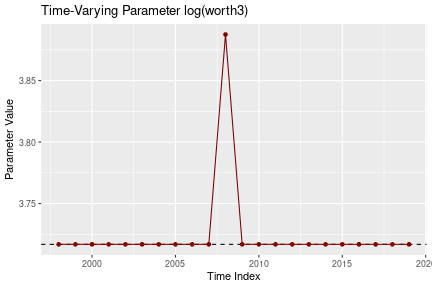Time-varying parameters of the Canada team based on the static model. plot(est_stnry)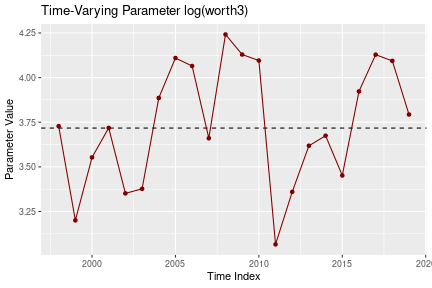Time-varying parameters of the Canada team based on the stationary model. plot(est_walk)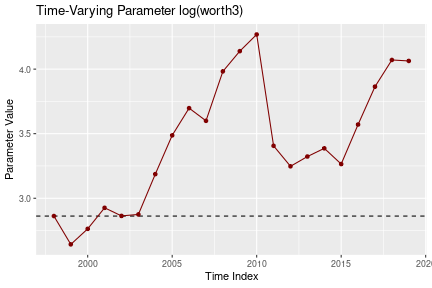Time-varying parameters of the Canada team based on the random walk model. However, it is important to note that these estimates are subject to uncertainty. To capture the uncertainty, we can utilize simulations by leveraging the gas_filter() function, which accepts the output of the gas() function as an argument. This allows us to obtain the standard deviations and quantiles for the worth parameter estimates, providing a more comprehensive understanding of the parameter dynamics over time. set.seed(42) flt_stnry <- gas_filter(est_stnry) To visualize time-varying parameters with confidence band, we can use the plot() on the gas_filter object. plot(flt_stnry)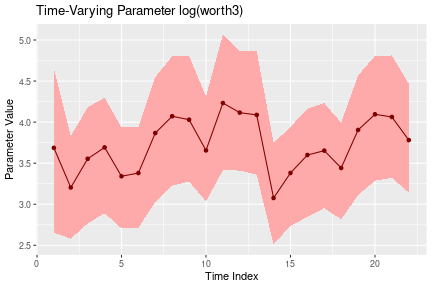Confidence band of time-varying parameters of the Canada team based on the stationary model. ## Forecasting Finally, we perform one-year-ahead forecasts. We use the gas_forecast() function, which can again take the estimated model as an argument. fcst_stnry <- gas_forecast(est_stnry, t_ahead = 1, x_ahead = 0) tibble(team = colnames(y)) %>% mutate(forecasted_strength = fcst_stnry$forecast$par_tv_ahead_mean[1, ]) %>% mutate(forecasted_gold = exp(forecasted_strength) / sum(exp(forecasted_strength))) %>% mutate(forecasted_rank = rank(-forecasted_strength)) %>% mutate(real_rank = ice_hockey_championships$rankings[24, ]) %>%
arrange(real_rank)
#> # A tibble: 24 × 5
#>    team  forecasted_strength forecasted_gold forecasted_rank real_rank
#>    <chr>               <dbl>           <dbl>           <dbl>     <dbl>
#>  1 CAN                 3.97        0.234                   2         1
#>  2 FIN                 3.97        0.235                   1         2
#>  3 USA                 2.09        0.0356                  6         3
#>  4 DEU                 0.742       0.00929                10         4
#>  5 RUS                 3.43        0.137                   3         5
#>  6 CHE                 1.82        0.0272                  7         6
#>  7 CZE                 3.41        0.134                   4         7
#>  8 SVK                 1.58        0.0214                  8         8
#>  9 SWE                 3.40        0.133                   5         9
#> 10 KAZ                -2.05        0.000569               19        10
#> 11 LVA                 0.978       0.0117                  9        11
#> 12 DNK                 0.229       0.00556                11        12
#> 13 NOR                 0.125       0.00501                12        13
#> 14 GBR                -3.55        0.000127               22        14
#> 15 BLR                -0.704       0.00219                14        15
#> 16 ITA                -0.957       0.00170                16        16
#> 17 AUT                -0.832       0.00192                15       Inf
#> 18 FRA                -0.490       0.00271                13       Inf
#> 19 HUN                -3.31        0.000162               21       Inf
#> 20 JPN                -2.21        0.000486               20       Inf
#> 21 KOR                -3.81        0.0000982              23       Inf
#> 22 POL                -3.99        0.0000821              24       Inf
#> 23 SVN                -1.95        0.000631               18       Inf
#> 24 UKR                -1.69        0.000813               17       Inf

The forecasted values can be displayed using the generic plot() function.

plot(fcst_stnry)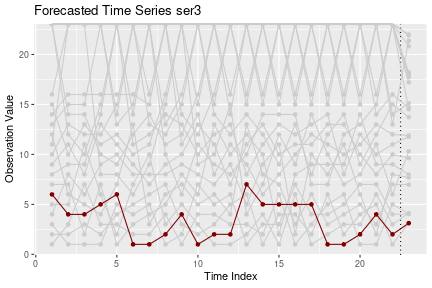One-step ahead forecasts of the Canada team based on the stationary model.